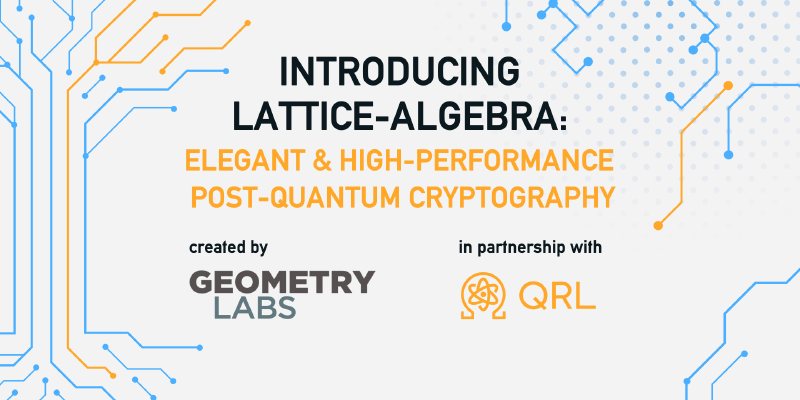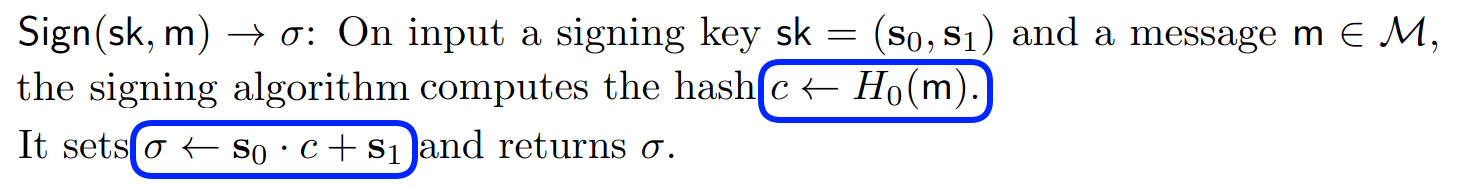# Introducing lattice-algebra: An elegant, high-performance post-quantum cryptography library

QRL, in partnership with Geometry Labs, are excited to announce a new lattice-algebra library for efficient, elegant, and high performance post-quantum secure cryptography.18th February 2022

We are excited to announce the public release of our python implementation of some of the algebra underlying lattice-based post-quantum cryptography. In partnership with The QRL Foundation and created by Geometry Labs, the code is now available as free and open source software published on GitHub and distributed through PyPi (install with: `pip install lattice-algebra`). This library will be used to prototype a variety of new features for the QRL protocol, such as lattice-based proof-of-stake signatures, trustless cross-chain atomic swaps (QRL↔BTC, QRL↔ETH, etc), and ‘lightning network’ style payment channels.

## Sharing the building blocks

The mathematical objects and calculations handled by this package are foundational for lattice algebra, with a variety of applications ranging from signature aggregation to zero-knowledge proofs. The module highly prioritizes developer experience for researchers and engineers, by allowing them to work with a few high level objects (e.g. polynomials, polynomial vectors) that contain built-in methods to abstractly handle the ways that they interact with each other. The goal is to lower the barrier for creating lattice cryptography primitives and applications by allowing the developers to focus on securely building the higher-level constructs without having to worry about implementing the underlying algebra as well.

The module is specifically designed for building cryptographic schemes in the Ring/Module/Ideal Short Integer Solution setting with secrets uniformly distributed with respect to the infinity-norm and one-norm; it can also be used to implement schemes in the Ring/Module/Ideal Learning With Errors setting. The library’s lead author Brandon Goodell explained how the high level objects are efficiently implemented under the hood, “to manipulate equations of polynomials, we carry out the computations with vectors and matrices, with highly optimized algebraic operations.

In this article series, we’ll show how the `lattice-algebra` library can be used to implement a Schnorr-like one-time signature scheme, an extension for non-interactive aggregatable one-time signatures, and adaptor signature functionality. Possible future applications include zero-knowledge arguments of knowledge, m-of-n multisig wallets with aggregatable signatures, and a python implementation of the NIST competitor CRYSTALS-Dilithium signature scheme.

## Mathematical background

In many lattice-based cryptographic schemes, primitives are constructed from polynomials in the ring `R = Zq[X]/(X^d + 1)` where we denote the integers modulo a prime `q` with `Zq`, with a degree`d` that is a power of two such that `(q-1) % (2*d) = 0`. Keys are often vectors from the vector space `V=R^l` or matrices with entries from `V = R^(k * l)` for dimensions `k`, `l`. For example, the CRYSTALS-Dilithium scheme sets `q = 2^23 - 2^13 + 1`, `d = 256`, and uses `4x4`, `6x5`, and `8x7` matrices, depending on security level.

For certain choices of `d`, `q`, and `l`, it is thought to be hard to find any vector (or matrix) `x` that is small enough (in terms of one or more norms on the ring `R`) such that some matrix equation `A * x = 0` is satisfied, where `A` is a suitably random challenge from `V`. From this hardness assumption, the map carrying suitably small vectors (or matrices) `x` to their images `A * x` is a one-way function. Simulation-based security proofs in the lattice setting are based on extracting a suitably small vector or matrix (called a witness) that satisfies some system of linear equations. Overall security of the scheme is based on how small the adversary can make this witness in terms of the norm of the witness.

The infinity-norm and the one-norm are of particular interest: the infinity-norm of a polynomial is the absolute maximum coefficient, and the one-norm is the absolute sum of coefficients. We can extend this definition to vectors by taking the maximum norm of the entries of the vector. We note that if we count only the weight of a polynomial, in terms of the number of non-zero coefficients, then we have that `one_norm <= infinity_norm * weight`. Consequently, bounding the infinity norm and the weight of a polynomial also has the effect of bounding the infinity norm and the one-norm. Taking into account both the infinity norm and the weight of the polynomial (number of non-zero entries) enables tighter inequalities that lead to smaller witnesses. This means we can achieve the same security level with smaller parameters (the CRYSTALS-Dilithium scheme is an exemplary implementation of this technique).

Nothing in `lattice-algebra` limits which hardness assumptions are underlying the cryptographic scheme being constructed. Since the library merely handles polynomials from `R` and vectors from `V=R^l`, schemes based on other hardness assumptions (such as the Ring Learning With Errors assumption) that take place over the same ring can be securely implemented as well.

## Designed for cryptography developers

The library is designed to make it easy for developers to write clean code that securely implements lattice-based cryptography for protocols and applications. The package is optimized to use the Number Theoretic Transform (NTT) to multiply polynomials in time `O(2dlog(2d))`, and uses constant-time modular arithmetic to avoid timing attacks. For convenience, we included tools for both hashing to and sampling from these “suitably small” polynomials and vectors. Both the hashing and sampling are carried out such that the bias of the resulting distribution is negligibly different from uniform.

One way that the `lattice-algebra` toolkit helps developers write succinct code is by leveraging python’s magic methods for arithmetic with elements from `R` and `R^l`. For example, suppose we have two polynomials `f` and `g`. Simple expressions such as `f + g`, `f - g`, and `f * g` carry out constant-time polynomial arithmetic such as addition, subtraction, and multiplication (respectively). Likewise if we have two vectors of polynomials `x` and `y`, several vector arithmetic methods are at our disposal: we can add them like `x + y`, or calculate the dot product as `x * y`. Additionally, `x ** f` scales a vector `x` by the polynomial `f`, which is useful for constructing digital signatures.

To showcase this style, consider the aggregatable signatures described in Boneh and Kim based on the signatures described by Lyubashevsky and Micciancio. Signing keys `sk = (s0, s1)` are pairs of elements sampled uniformly from the subset of `R^l` with bounded norm. The signature challenge `c` is computed from a hash function `H_0` that digests a message `m` and outputs an element in `R` with bounded norm.

Here is how the process is described in the Boneh and Kim write up:With proper parameters and key structure, we can implement a functioning “arithmetic-y” version of `sign` simply by transcribing the above blue boxes:

 ``````1 2 3 `````` ``````def sign(sk: Tuple[PolynomialVector, PolynomialVector], m: str) -> PolynomialVector: c: Polynomial = H_0(m) return sk ** c + sk ``````

The ability to write such minimalist code limits the room for implementation errors and allows cryptography developers (and their code reviewers) to focus on the application or protocol itself.

## Future work

Future work on the `lattice-algebra` package includes non-uniform key sampling, expanded linear algebra, and additional benchmarking tests. We will shortly be publishing articles with free open-source code for several cryptographic applications, including aggregatable signatures and adaptor signatures (which will be used to prototype the logic enabling cross-chain atomic swaps and ‘lightning network’ style payment channels for the QRL protocol).

## Library Contributors

Brandon Goodell (lead author), Mitchell P. Krawiec-Thayer, Rob Cannon

Correspondence: info@geometrylabs.io

18th February 2022